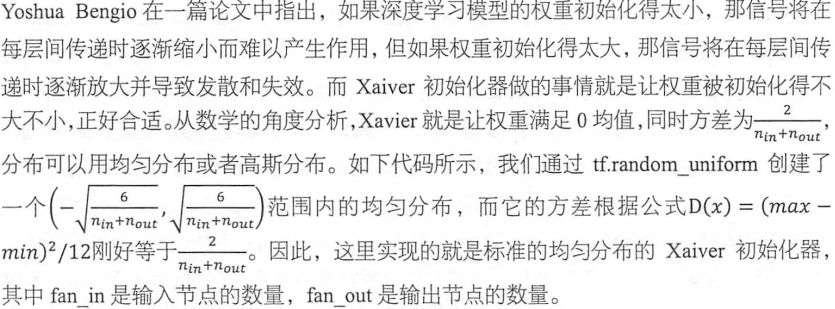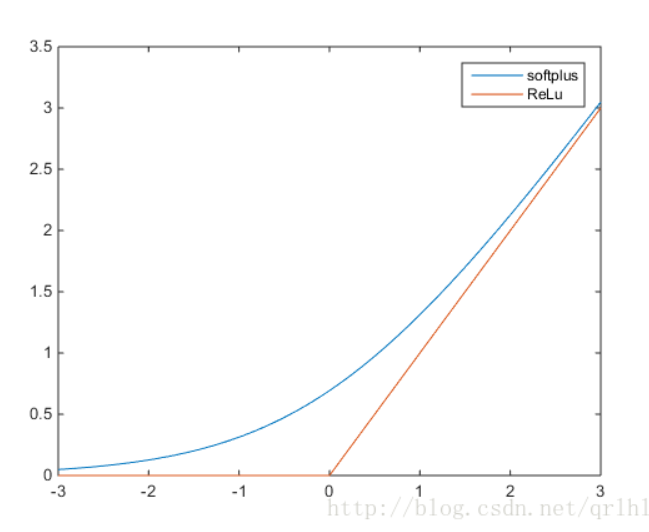# TensorFlow实战——学习笔记（一）去噪自编码器实现

## 导入模块和数据

import numpy as np
import sklearn.preprocessing as prep
import tensorflow as tf
from tensorflow.examples.tutorials.mnist import input_data

class AdditiveGaussianNoiseAutoencoder(object):
def __init__(self, n_input, n_hidden, transfer_function=tf.nn.softplus,
self.n_input = n_input
self.n_hidden = n_hidden
self.transfer = transfer_function
self.scale = tf.placeholder(tf.float32)
self.training_scale = scale
network_weights = self._initialize_weights()
self.weights = network_weights

        self.x = tf.placeholder(tf.float32, [None, self.n_input])
self.hidden = self.transfer(tf.add(tf.matmul(self.x + scale * tf.random_normal((n_input,)),
self.weights["w1"]), self.weights["b1"]))
self.reconstruction = tf.add(tf.matmul(self.hidden, self.weights["w2"]),
self.weights["b2"])

        self.cost = 0.5 * tf.reduce_sum(tf.pow(tf.subtract(self.reconstruction, self.x), 2.0))
self.optimizer = optimizer.minimize(self.cost)

init = tf.global_variables_initializer()
self.sess = tf.Session()
self.sess.run(init)

    def _initialize_weights(self):
all_weights = dict()
all_weights["w1"] = tf.Variable(xavier_init(self.n_input, self.n_hidden))
all_weights["b1"] = tf.Variable(tf.zeros([self.n_hidden], dtype=tf.float32))
all_weights["w2"] = tf.Variable(tf.zeros([self.n_hidden, self.n_input], dtype=tf.float32))
all_weights["b2"] = tf.Variable(tf.zeros([self.n_input], dtype=tf.float32))
return all_weights

    def partial_fit(self, X):
cost, opt = self.sess.run((self.cost, self.optimizer),
feed_dict={self.x: X,
self.scale: self.training_scale})
return cost

    def calc_total_cost(self, X):
return self.sess.run(self.cost,
feed_dict={self.x: X, self.scale: self.training_scale})


    def transform(self, X):
return self.sess.run(self.hidden,
feed_dict={self.x: X, self.scale: self.training_scale})

    def generate(self, hidden=None):
if hidden is None:
hidden = np.random.normal(size=self.weights["w1"])
return self.sess.run(self.reconstruction, feed_dict={self.hidden: hidden})

    def reconstruct(self, X):
return self.sess.run(self.reconstruction,
feed_dict={self.x: X, self.scale: self.training_scale})

    def get_weights(self):
return self.sess.run(self.weights['w1'])

    def get_biases(self):
return self.sess.run(self.weights["b1"])

def standard_scale(X_train, X_test):
preprocessor = prep.StandardScaler().fit(X_train)
X_train = preprocessor.transform(X_train)
X_test = preprocessor.transform(X_test)
return X_train, X_test

def get_random_block_from_data(data, batch_size):
start_index = np.random.randint(0, len(data) - batch_size)
return data[start_index: (start_index + batch_size)]
def xavier_init(fan_in, fan_out, constant=1):
low = -constant * np.sqrt(6.0 / (fan_in + fan_out))
high = constant * np.sqrt(6.0 / (fan_in + fan_out))
return tf.random_uniform((fan_in, fan_out),
minval=low, maxval=high,
dtype=tf.float32)
if __name__ == '__main__':
# 加载数据
mnist = input_data.read_data_sets('../datas/MNIST_data/', one_hot=True)
# 对数据进行标准化变换
X_train, X_test = standard_scale(mnist.train.images, mnist.test.images)
# 定义几个常用参数：总训练样本数，最大训练轮数（epochs），batch_size，设置每隔一轮就显示一次损失cost
n_samples = int(mnist.train.num_examples)
training_epochs = 20
batch_size = 128
display_step = 1

n_input=784,
n_hidden=200,
transfer_function=tf.nn.softplus,
scale=0.01
)

for epoch in range(training_epochs):
avg_cost = 0.
total_batch = int(n_samples / batch_size)
for i in range(total_batch):
batch_xs = get_random_block_from_data(X_train, batch_size)

cost = autoencoder.partial_fit(batch_xs)
avg_cost += cost / n_samples * batch_size

if epoch % display_step == 0:
print("Epoch: %04d" % (epoch + 1), "cost={:.9f}".format(avg_cost))

print("Total cost:" + str(autoencoder.calc_total_cost(X_test)))

# 实现过程中遇到的几个问题：

### 1、为什么要初始化参数？### 2、tf.subtract函数的原理，还有tf.pow()

subtract(x ，y ，name=None)，向量减法
pow(x ，y ，name=None),计算x的y次方（mi）

### 3、为什么对训练，测试数据进行标准化处理

#### 什么时候对数据中心化呢

1、在聚类过程中，标准化显得尤为重要。这是因为聚类操作依赖于对类间距离和类内聚类之间的衡量。如果一个变量的衡量标准高于其他变量，那么我们使用的任何衡量标准都将受到该变量的过度影响。

2、在PCA降维操作之前。在主成分PCA分析之前，对变量进行标准化至关重要。 这是因为PCA给那些方差较高的变量比那些方差非常小的变量赋予更多的权重。而 标准化原始数据会产生相同的方差，因此高权重不会分配给具有较高方差的变量

3、KNN操作，原因类似于kmeans聚类。由于KNN需要用欧式距离去度量。标准化会让变量之间起着相同的作用。

4、在SVM中，使用所有跟距离计算相关的的kernel都需要对数据进行标准化。

5、在选择岭回归和Lasso时候，标准化是必须的。原因是正则化是有偏估计，会对权重进行惩罚。在量纲不同的情况，正则化会带来更大的偏差。

### 5、tf.random_uniform的原理

random_uniform(
shape,
minval=0,
maxval=None,
dtype=tf.float32,
seed=None,
name=None
)

### 6、为什么使用softplus

ReLu函数的全称为Rectified Linear Units，函数表达式为y=max(0,x)，softplus函数的数学表达式为y=log(1+e^x)，它们的函数表达式如下: